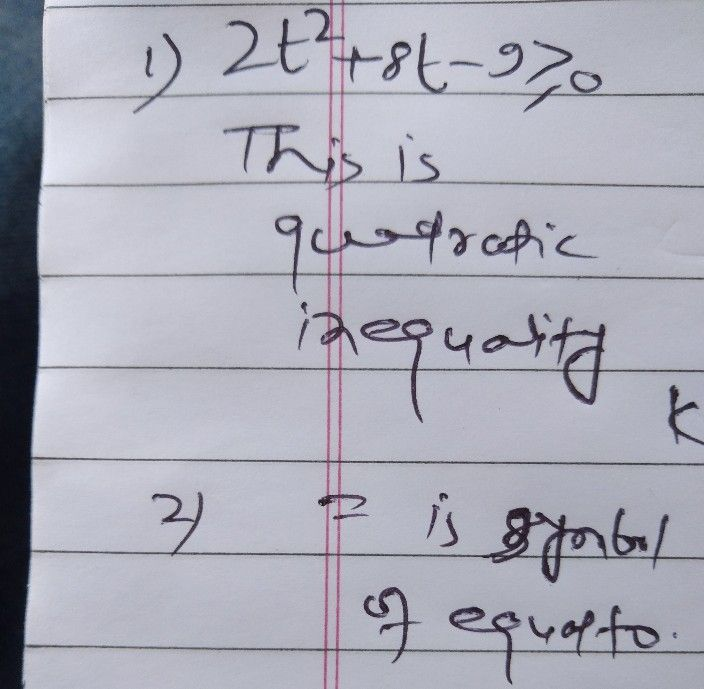Symbol
Problem$11$ $1$ Which of the following mathematical statements is inequality? $A$ $5r^{2}-4r-5=0$ - $\square$ $3y+12<0$ $C$ $a90a0ra0cn$ $2t^{2}+8t-9\geq 0$ $\square$ $s^{3}+8s+15$ # 0 $21$ $T1n0$ following are $symbols$ used in inequalities $xco0$ $A3$ $\leq$ $B3$ > C. = D. 2 $3$ 3. Which of the following coordinates of points belong to the solution set of the inequality
7th-9th grade
Other
Search count: 115
SolutionQanda teacher - jaisi